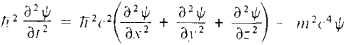# Klein-Gordon Equation

Also found in: Wikipedia.

## Klein-Gordon equation

[′klīn ′gȯrd·ən i‚kwā·zhən]
(quantum mechanics)
A wave equation describing a spinless particle which is consistent with the special theory of relativity. Also known as Schrödinger-Klein-Gordon equation.
McGraw-Hill Dictionary of Scientific & Technical Terms, 6E, Copyright © 2003 by The McGraw-Hill Companies, Inc.
The following article is from The Great Soviet Encyclopedia (1979). It might be outdated or ideologically biased.

## Klein-Gordon Equation

a relativistic (that is satisfying the requirements of the theory of relativity) quantum equation for particles with zero spin.

Historically, the Klein-Gordon equation was the first relativistic equation in quantum mechanics for the wave function ψ of a particle. It was proposed in 1926 by E. Schrödinger as a relativistic generalization of the Schrödinger equation. It was also proposed, independently, by the Swedish physicist O. Klein, the Soviet physicist V. A. Fok, and the German physicist W. Gordon.

For a free particle, the Klein-Gordon equation is writtenThis equation is associated with the relativistic relationship between the energy £ and the momentum p of a particle £ 2 = p2c2 + m2c4, where m is the mass of the particle and c is the speed of light.

The solution to the equation is the function ψ (x, y, z, t), which is a function only of the coordinates (x, y, z) and the time (t). Consequently, the particles described by this function have no other internal degrees of freedom—that is, they are spinless (the π-meson and the K-meson are of this type). However, analysis of the equation indicated that its solution ψ differed fundamentally in physical meaning from the ordinary wave function, which is considered as the probability amplitude of detecting a particle at a given point in space and a given moment in time: ψ(x, y, z, f) is not determined uniquely by the value of ψ at the initial moment (such an unambiguous relationship is postulated in nonrelativistic quantum mechanics).

Furthermore, the expression for the probability of a given state can take on not only positive values but also negative values, which are devoid of physical meaning. Therefore, the Klein-Gordon equation was at first rejected. However, in 1934, W. Pauli and W. Weisskopf discovered a suitable interpretation for the equation within the scope of quantum field theory; treating it like a field equation analogous to Maxwell’s equations for an electromagnetic field, they quantized it, so that ψ became an operator.

M. A. LIBERMAN

References in periodicals archive ?
Recently, this technique was also established in the numerical analysis of low-regularity problems [21, 28] and introduced for the highly oscillatory Klein-Gordon equation in .
Ntamack, "Solutions of the Klein-Gordon Equation with the Hulthen Potential Using the Frobenius Method," International Journal of Theoretical and Mathematical Physics, vol.
Wainger, "Lp-Lq estimates for the Klein-Gordon equation," Journal de Mathematiques Pures et Appliquees, vol.
Earlier version of HAM methods has been applied for various nonlinear problem solving such as the Klein-Gordon equation , El Nino Southern Oscillation , Huxley , Zakharov-Kuznetsov equation , and one species growth model in the polluted environment .
1, Klein formulated in his paper (5) (1927) the general method to treat the interaction between charged particles and an electromagnetic field on the basis of the semiclassical treatment; hence he should be called the founder of the semi-classical treatment (the Klein-Gordon equation was also derived here).
Another well-known model, time fractional nonlinear Klein-Gordon equation with proportional delay, aries in quantum field theory to describe nonlinear wave interaction:
Consider the one-dimensional linear inhomogeneous Klein-Gordon equation
Several studies were carried out for the numerical approximation of the second order linear Klein-Gordon equation. This study constructed a new numerical technique for the numerical approximation of second order linear Klein-Gordon equation.
for fermions, we get the Klein-Gordon equation and Dirac equation
The proof is standard and follows for example  (see also  in the context of the Klein-Gordon equation).
Bogolyubskii, "Oscillating particle-like solutions of the nonlinear Klein-Gordon equation," JETP Letters, vol.
(15) as well as its shape closely resemble those of the X wave solution to the Klein-Gordon equation, and for [[omega].sub.p] = 0 the X wave solution of the scalar wave equation in free space.

Site: Follow: Share:
Open / Close